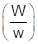# 20%of compound-A in benzene has same boiling point as 45%of compound-B in same solvent. If molar mass of A is 20 g mol–1 then molar mass of B is (compound-A is non-electrolyte but compound-B undergoes 100% dimerization at the given concentration).

one year ago

Let the total mass of the solution be 100 g and the mass of benzene be 30 g.

∴ Mass of carbon tetrachloride = (100 - 30)g = 70 g

Molar mass of benzene (C6H6) = (6 × 12 + 6 × 1) g mol - 1

= 78 g mol - 1

∴ Number of moles of C6H6  =30/78 mol

= 0.3846 mol

Molar mass of carbon tetrachloride (CCl4) = 1 × 12 + 4 × 35.5

= 154 g mol - 1

∴ Number of moles of CCl4 = 70/154 mol

= 0.4545 mol

Thus, the mole fraction of C6H6 is given as:

= 0.3846 / (0.3846 +0.4545)

= 0.458